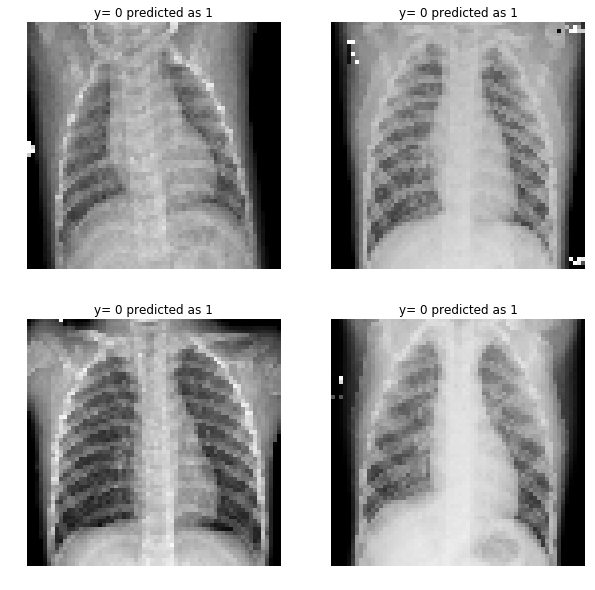# Classification of Chest X-Ray Image Part 2¶

### Elan Ding¶

Modified: July 11, 2018

We are going to use the algorithm from the previous post and train a simple 2-layer neural network on the chest x-ray data. I have compiled all the code into a package my_package.

In :
import numpy as np
import matplotlib.pyplot as plt
from my_package.deep_neural_network import *


In :
data = np.load('xray_data.npy')
train_x = data
train_y = data
valid_x = data
valid_y = data
test_x = data
test_y = data

print('Training data has dimension: {}'.format(train_x.shape))
print('Training label has dimension: {}'.format(train_y.shape))
print('Validation data has dimension: {}'.format(valid_x.shape))
print('Validation label has dimension: {}'.format(valid_y.shape))
print('Test data has dimension: {}'.format(test_x.shape))
print('Test label has dimension: {}'.format(test_y.shape))

Training data has dimension: (12288, 5216)
Training label has dimension: (1, 5216)
Validation data has dimension: (12288, 16)
Validation label has dimension: (1, 16)
Test data has dimension: (12288, 624)
Test label has dimension: (1, 624)


Our 2-layer neural network will have a hidden layer consisting of 7 nodes and an output layer.

In :
layers_dims = (12288, 7, 1)


We run 2500 steps of gradient descent and store the output in the variable parameters.

In :
parameters = deep_model(train_x, train_y, layers_dims = layers_dims, num_iterations = 2500, print_cost=True)

Cost after iteration 0: 0.694293
Cost after iteration 100: 0.551729
Cost after iteration 200: 0.470766
Cost after iteration 300: 0.350222
Cost after iteration 400: 0.256993
Cost after iteration 500: 0.207847
Cost after iteration 600: 0.179617
Cost after iteration 700: 0.161295
Cost after iteration 800: 0.148639
Cost after iteration 900: 0.139493
Cost after iteration 1000: 0.132640
Cost after iteration 1100: 0.127329
Cost after iteration 1200: 0.123079
Cost after iteration 1300: 0.119575
Cost after iteration 1400: 0.116609
Cost after iteration 1500: 0.114037
Cost after iteration 1600: 0.111763
Cost after iteration 1700: 0.109716
Cost after iteration 1800: 0.107848
Cost after iteration 1900: 0.106122
Cost after iteration 2000: 0.104511
Cost after iteration 2100: 0.102996
Cost after iteration 2200: 0.101560
Cost after iteration 2300: 0.100192
Cost after iteration 2400: 0.098882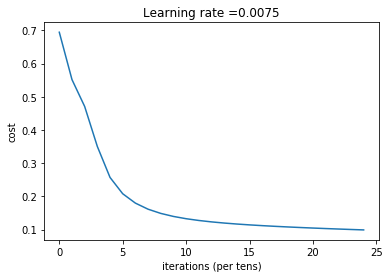Let's define a function that predicts whether an x-ray image is pneunomia or normal based on the output probabilities. It also gives the total accuracy as the percentage of total matched pairs between predicted results and actual results.

In :
def predict(X, y, parameters):

m = X.shape
n = len(parameters) // 2
p = np.zeros((1,m))

probs, caches = model_forward(X, parameters)

for i in range(0, probs.shape):
if probs[0,i] > 0.5:
p[0,i] = 1
else:
p[0,i] = 0

print("Accuracy: "  + str(np.sum((p == y)/m)))

return p.astype(int), probs

In :
predictions_train, probs_train = predict(train_x, train_y, parameters)

Accuracy: 0.9647239263803681

In :
predictions_test, probs_test  = predict(test_x, test_y, parameters)

Accuracy: 0.7467948717948718

In :
from sklearn.metrics import classification_report
print(classification_report(np.squeeze(test_y), np.squeeze(predictions_test)))

             precision    recall  f1-score   support

0       0.94      0.35      0.51       234
1       0.72      0.99      0.83       390

avg / total       0.80      0.75      0.71       624



This is very interesting! The precision and recall is exactly the same as logistic regression. Let's take a look at the ROC curve.

In :
from sklearn.metrics import roc_curve, auc

fpr, tpr, _ = roc_curve(np.squeeze(test_y), np.squeeze(probs_test))
roc_auc = auc(fpr, tpr)

plt.figure()
lw = 2
plt.plot(fpr, tpr, color='darkorange',
lw=lw, label='ROC curve (area = %0.2f)' % roc_auc)
plt.plot([0, 1], [0, 1], color='navy', lw=lw, linestyle='--')
plt.xlim([0.0, 1.0])
plt.ylim([0.0, 1.05])
plt.xlabel('False Positive Rate')
plt.ylabel('True Positive Rate')
plt.legend(loc="lower right")
plt.show()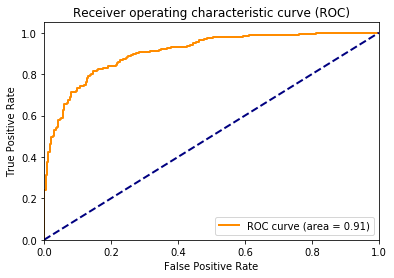We get AUC of 0.91, which is only 1% above logistic regression! Let's take a look at the images that are mis-classified.

In :
from pathlib import Path
import glob
import pandas as pd
import cv2

In :
data_dir = Path('chest_xray')

train_dir = data_dir / 'train'
val_dir = data_dir / 'val'
test_dir = data_dir / 'test'

In :
normal_cases_dir = test_dir / 'NORMAL'
pneumonia_cases_dir = test_dir / 'PNEUMONIA'

normal_cases = normal_cases_dir.glob('*.jpeg')
pneumonia_cases = pneumonia_cases_dir.glob('*.jpeg')

test_data = []

for img in normal_cases:
test_data.append((img,0))

for img in pneumonia_cases:
test_data.append((img, 1))

test_data = pd.DataFrame(test_data, columns=['image', 'label'],index=None)


Here is an example of the picture that is mis-classified.

In :
# Example of a mis-classified picture
index = 203
print ("y = " + str(test_y[0, index]) + ", you predicted that it is a \"" + str(predictions_test[0, index]))

y = 0, you predicted that it is a "1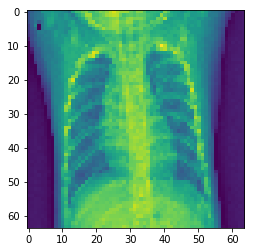Let's take a look at some more false negative examples. Note that since the recall is 99%, the false negative is very rare. If the model predicts negative, it is most likely to be correct.

In :
index = test_data[(np.squeeze(test_y) != np.squeeze(predictions_test))
& (np.squeeze(test_y) == 1)].index
index = np.array(index)[0:4]

In :
# false negatives
f, ax = plt.subplots(2,2, figsize=(10,10))

for i in range(4):
img = cv2.resize(img, (64, 64))
ax[i//2, i%2].imshow(img, cmap='gray')
ax[i//2, i%2].set_title("y= " + str(test_y[0, index[i]]) +
" predicted as " + str(predictions_test[0, index[i]])
)
ax[i//2, i%2].axis('off')
ax[i//2, i%2].set_aspect('auto')
plt.show()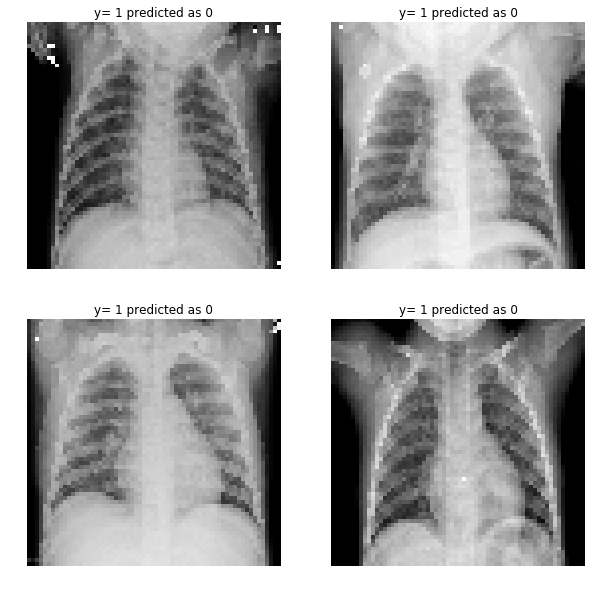Finally we show some false positive examples. False positive rate is very high so we have a lot of these examples.

In :
index = test_data[(np.squeeze(test_y) != np.squeeze(predictions_test))
& (np.squeeze(test_y) == 0)].index
index = np.array(index)[0:4]

In :
# false positives
f, ax = plt.subplots(2,2, figsize=(10,10))

for i in range(4):